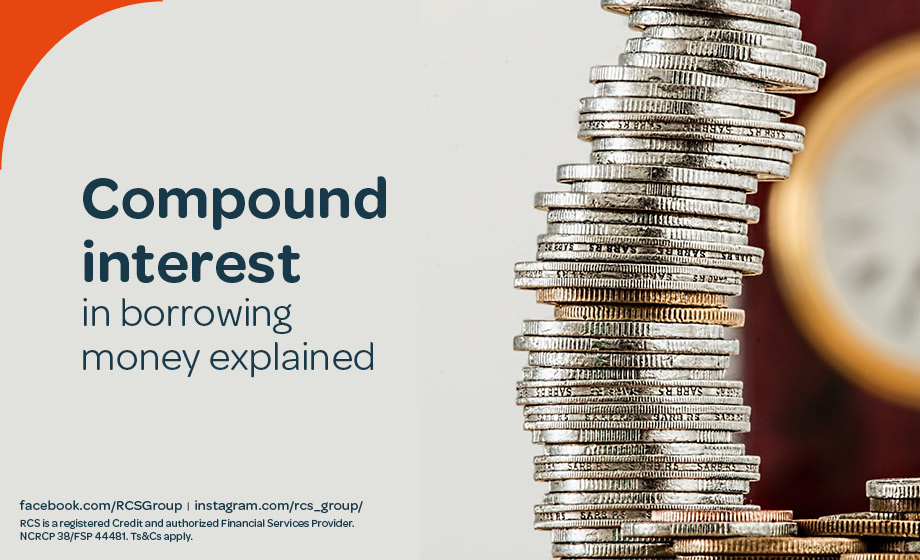# Compound Interest Explained

11 AUGUST 2023

Interest plays an important part in borrowing money and understanding how the different varieties are calculated is key. This article will explain what compound interest is and how to calculate it.## What is compound interest?

Compound interest is the interest on a loan or investment that is calculated on the original principal amount as well as previously accumulated interest. This means that the rate at which interest grows increases over time. The interest percentage will stay the same but the amount of interest added gets higher after each compounding. Compound interest thus allows for exponential growth.

Depending on your loan agreement, interest can be compounded at different rates from daily, monthly, or annually. The more interest compounds on your loan, the faster the principal will grow and the more interest will be added to what you need to pay, overall. Understand how frequently your interest will be compounded before signing an agreement.

## What types of loans use compound interest?

Credit card loans and student loans are loans most likely to use compound interest. Your monthly repayments are amortized, meaning that with each payment you are paying off your principal amount as well as the interest. Set up a payment schedule to make sure that this is being done and to find out how often the interest will be compounded.

For home loans, personal loans, business loans, and credit card loans, the compounding schedule is typically every month. Some banks also offer continuously compounding interest, adding interest to your principal amount at every possible chance. This, however, does not accumulate much more than daily compounding interest if you are not depositing or withdrawing money on the same day. This type of frequent compounding benefits investors and creditors more than borrowers.

## How do you calculate compound interest?

The formula for compound interest as well as the principal sum is:

A = P (1 + r/n) (nt)

• A = the value of the investment or loan including interest in the future
• P = the principal amount, being the initial deposit or loan amount
• r = the annual decimal interest rate
• n = the number of times that interest is compounded per t unit
• t = the loan term or time the money is invested for

The above formula calculates the total value of principal and interest in the future. To calculate the compound interest only, you will need to subtract the principal amount from the result of the above formula. The formula for compound interest only is:

P (1 + r/n) (nt) - P

For example, the total value of a principal amount of R5,000 deposited into a savings account at an annual interest rate of 15%, compounded monthly, after 10 years can be calculated as;

• P = 5000
• r = 0.15
• n = 12
• t = 10

A = 5000 (1 + 0.15 / 12) (12 * 10) = 8235.05

This means the investment balance after 10 years is R22,201.07. Or the total amount you will have paid off after a loan period of 10 years on a loan of R5,000.

Make use of the many online compound interest calculators to help you, or even use Microsoft Excel. This will help you when making a decision regarding the details of loan agreements.

## Compound Interest vs. Accrued Interest vs. Simple Interest

Accrued interest is interest that has been accumulated over a period of time but has not been paid or credited yet. It is commonly used when fixed-income investment accumulates a stable amount of interest such as bonds, which can be paid out over a set short period of time. This differs from compound interest, as interest accumulated is regularly credited.

Simple interest is the most basic form of interest that is only calculated on the principal amount and not accumulated interest. This is the best interest to have on a loan as you would only be paying interest on a smaller amount and is a set amount that does not change over time.

## How can you earn on compound interest?

As compound interest increases the amount of interest you earn unlike simple interest, it is a good way to earn money on investments or savings. Most saving accounts have compounding interest, however they may have lower interest rates. This means that you would need to make a deposit of a larger amount of money or wait for a longer period of time to receive returns.

If you are taking a loan out on compound interest, expect higher costs than if it were simple interest. Make sure that when you compare loans, you compare their APR which will give you an overall cost of the loan along with all fees. Now that you know how to calculate compound interest, choose a loan that will best suit your needs and financial circumstance.